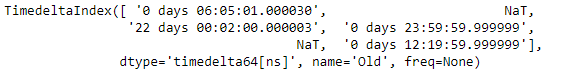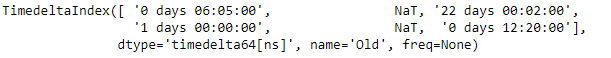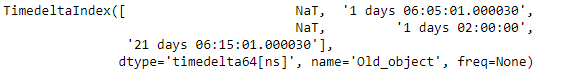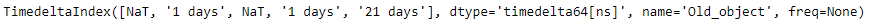# Python | Pandas TimedeltaIndex.round

• Last Updated : 06 Jan, 2019

Python is a great language for doing data analysis, primarily because of the fantastic ecosystem of data-centric python packages. Pandas is one of those packages and makes importing and analyzing data much easier.

Pandas` TimedeltaIndex.round()` function round the labels of the given TimedeltaIndex object to the specified frequency.

Syntax : TimedeltaIndex.round(freq, *args, **kwargs)

Parameters :
freq : freq string/object

Return : index of same type

Example #1: Use `TimedeltaIndex.round()` function to round the labels of the given TimedeltaIndex object.

 `# importing pandas as pd``import` `pandas as pd`` ` `# Create the TimedeltaIndex object``tidx ``=` `pd.TimedeltaIndex(data ``=``[``'06:05:01.000030'``, ``None``, ``'22 day 2 min 3us 10ns'``,``                     ``'+23:59:59.999999'``, ``None``, ``'+12:19:59.999999'``], name ``=``'Old'``)`` ` `# Print the TimedeltaIndex object``print``(tidx)`

Output :Now we will use the `TimedeltaIndex.round()` function to round the labels of the tidx object to minutely frequency.

 `# round the labels of the tidx``# object to minutely frequency``tidx.``round``(``'T'``)`

Output :As we can see in the output, the `TimedeltaIndex.round()` function has rounded off the value of the given TimedeltaIndex object to the desired frequency.

Example #2: Use `TimedeltaIndex.round()` function to rename the given TimedeltaIndex object.

 `# importing pandas as pd``import` `pandas as pd`` ` `# Create the TimedeltaIndex object``tidx ``=` `pd.TimedeltaIndex(data ``=``[``None``, ``'1 days 06:05:01.000030'``, ``None``,``                                ``'1 days 02:00:00'``, ``'21 days 06:15:01.000030'``],``                                                           ``name ``=``'Old_object'``)`` ` `# Print the TimedeltaIndex object``print``(tidx)`

Output :Now we will use the `TimedeltaIndex.round()` function to round the labels of the tidx object to daily frequency.

 `# round the labels of the tidx ``# object to daily frequency``tidx.``round``(``'D'``)`

Output :As we can see in the output, the `TimedeltaIndex.round()` function has rounded off the value of the given TimedeltaIndex object to the desired frequency.

My Personal Notes arrow_drop_up